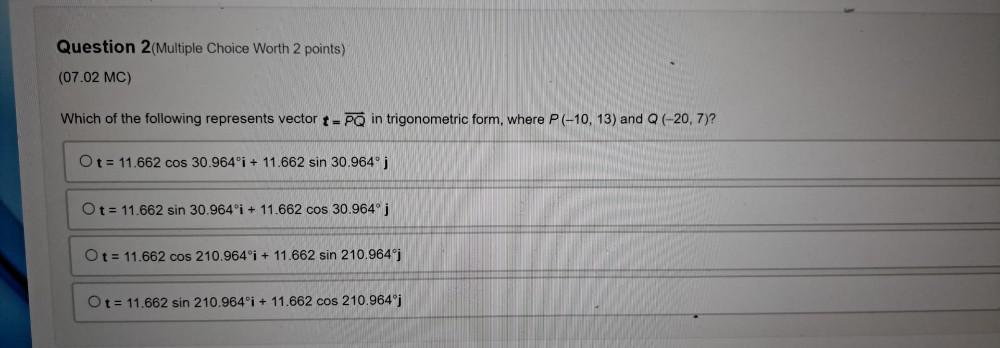Question:

# Which of the following represents vector t = PQ in trigonometric form, where P(-10, 13) and Q (-20, 7)? O t = 11.662 cos 30.964°i + 11.662 sin 30.964°j ; O t= 11.662 sin 30.964°i + 11.662 cos 30.964°Which of the following represents vector t = PQ in trigonometric form, where P(-10, 13) and Q (-20, 7)? O t = 11.662 cos 30.964°i + 11.662 sin 30.964°j ; O t= 11.662 sin 30.964°i + 11.662 cos 30.964° j O t= 11.662 cos 210.964°i + 11.662 sin 210.964ºj O t= 11.662 sin 210.964°i + 11.662 cos 210.964°j